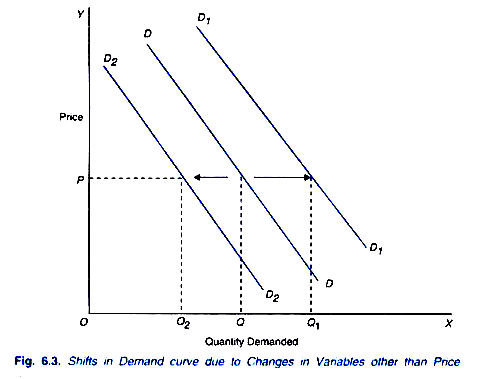# Relationship between Demand Function and Demand Curve

It is important to know the relationship between demand function and demand curve.

While a complete demand function specifies the relationship between quantity demanded of a product and many variables such as the own price of the product, income of consumers prices of related commodities, tastes and preferences, expected future prices etc.

(Qxd )= f (Px, I, Px, T, A, N) the demand curve is a graphic representation of only a part of this demand function, namely, Qxd = f(P), holding other independent variables in the demand function as constant.

That is, while drawing a demand curve values of other variables in the demand function are kept constant at particular levels. The complete demand function with many variables cannot be shown by the two dimensional curve, the effect of changes in other variables or factors on the quantity demanded of a product is shown by shifts in the whole demand curve. This is illustrated in Figure 6.3. To begin with DD is the market demand curve.Now, suppose income of consumers increases, say due to the hike in their wages and salaries. As a result, they will demand more of a product at each price causing a shift in the demand curve to the right as from DD to D1D1 in Figure 6.3.

It will be seen that at a given price OP, with demand curve DD the consumers were buying OQ quantity of the product and with rightward shift in the demand curve to D1D1 they demand greater quantity OQ1 of the product. Similarly, at other prices more is demanded at D1D1 as compared to the demand curve DD.